# How to calculate quarter start date or end date based on a given date in Excel?

If you manage a business or are involved in almost any other field, it is quite likely that you will often find yourself in a situation in which you need to determine which calendar quarter a certain date falls under. Excel does not come equipped with a feature that can generate this information based on a certain date, which is a great disappointment. By using this strategy, we are able to convert any given day into a calendar quarter.

If you already have a column of dates and you want to achieve the following result, you will need to modify the dates so that they only include the beginning date and the ending date of the quarter.

## Step 1

In The following is a simple formula that you may use to determine the beginning and ending dates of the quarter depending on the date that was provided to you. To do so, kindly follow the guidelines that are listed below.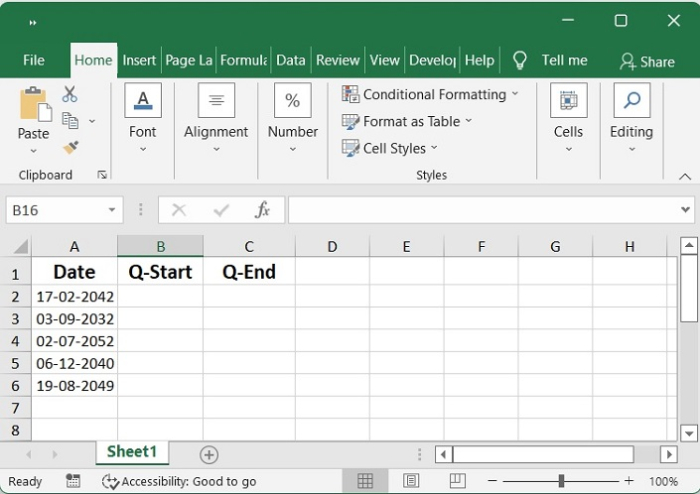## Step 2

After inserting the following formula into an empty cell at the point where you desire to output the calculated result, the date will be displayed in a way that takes into consideration both the date the quarter started as well as the date the current quarter began.; this will result in all of the start dates of the quarter being computed according to the specified dates.

=DATE(YEAR(A2),FLOOR(MONTH(A2)-1,3)+1,1)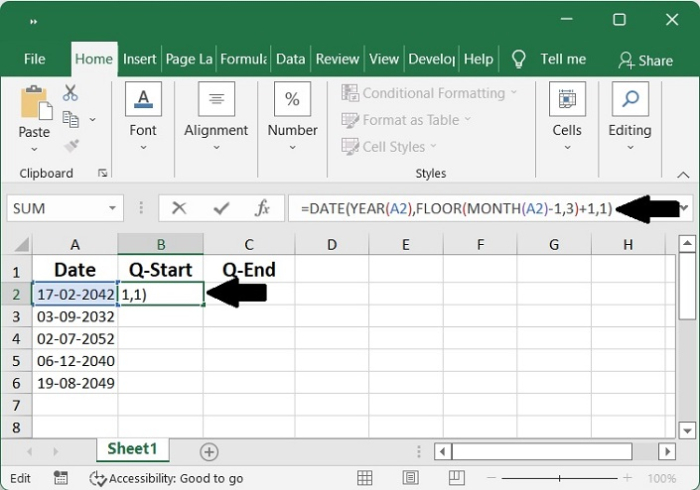## Step 3

In order to put this formula into action, you will need to drag the fill handle all the way down until it is touching the cells.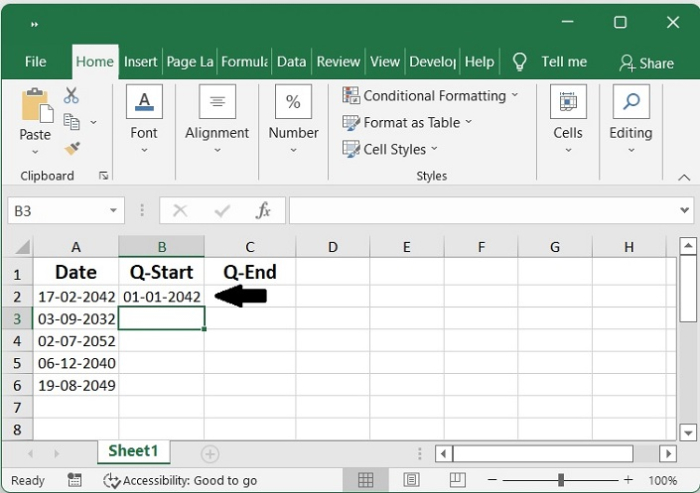## Step 4

After inserting the following formula into an empty cell at the point where you desire to output the calculated result, the date will be displayed in a way that takes into consideration both the date the quarter ended as well as the date the current quarter end.; this will result in all of the end dates of the quarter being computed according to the specified dates.

=DATE(YEAR(A2),((INT((MONTH(A2)-1)/3)+1)*3)+1,1)-1


Using a given date as the starting point for calculating the end date of a quarter,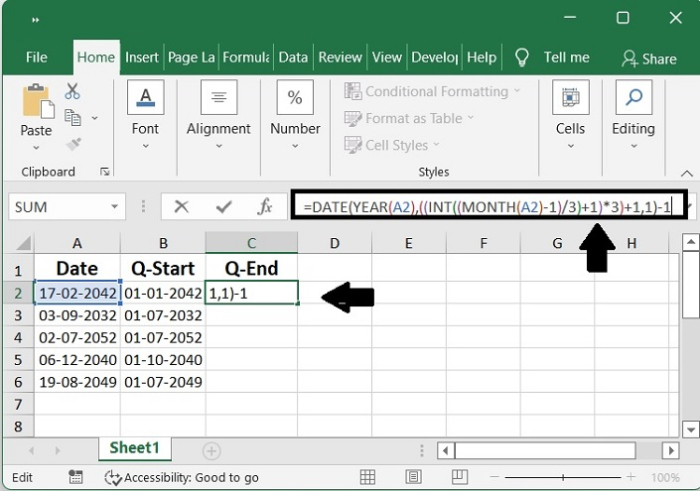## Step 5

You may apply this formula to the cells that you desire by dragging the fill handle down to those cells, and it will compute all the end dates of the quarter based on the dates that were supplied.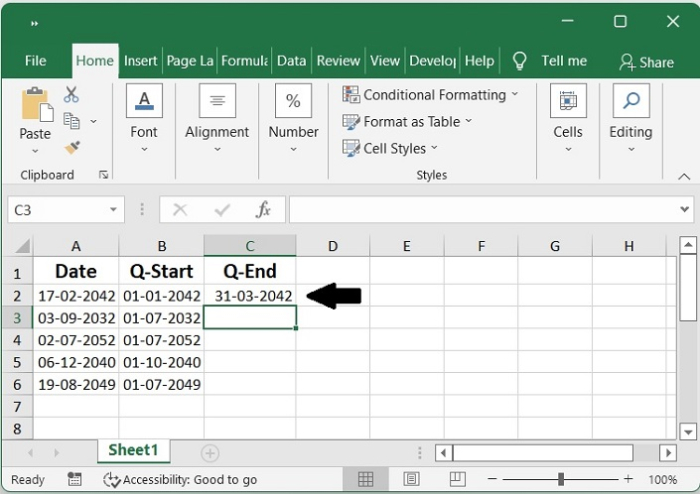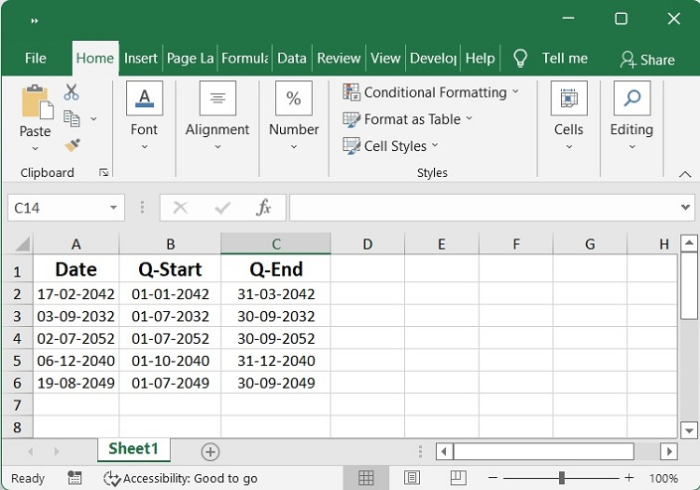## Conclusion

In this tutorial, we used a simple example to demonstrate how you can calculate the start and end data based on a given date in Excel.